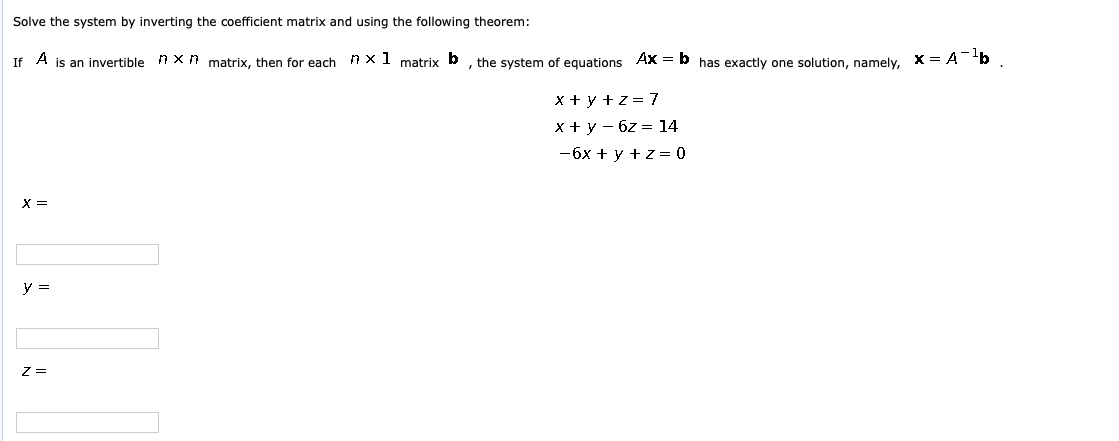Home / Answered Questions / Other / solve-the-system-by-inverting-the-coefficient-matrix-and-using-the-following-theorem-if-a-is-an-inve-aw575

# (Solved): Solve The System By Inverting The Coefficient Matrix And Using The Following Theorem: If A Is An Inv...Solve the system by inverting the coefficient matrix and using the following theorem: If A is an invertible mxn matrix, then for each mxl matrix b , the system of equations Ax = b has exactly one solution, namely, X= A b. x + y + z=7 x + y - 62 = 14 -6x + y + z=0 X = y = Z=

We have an Answer from Expert